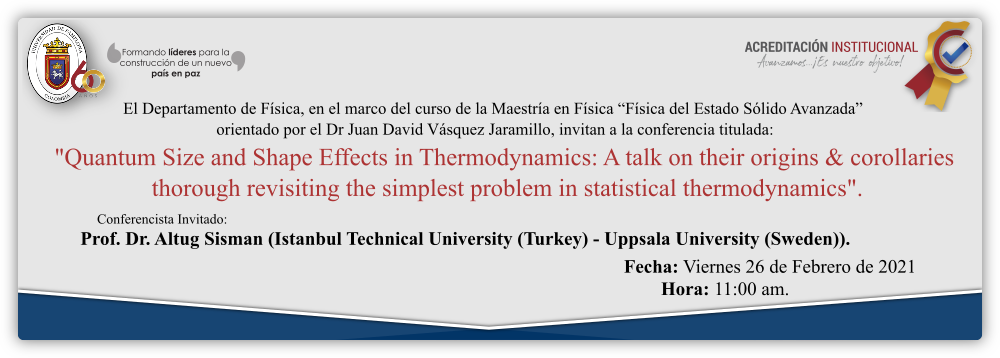# ConferenciasABSTRACT

Nanoscale thermodynamics became one of the most interesting topics of thermodynamics especially in last two decades. Fundamental expressions of thermodynamic quantities are based on infinite summations over either momentum or energy eigenvalues. For macroscopic systems, we assume that the system is unbounded (infinitely large, classical limit) and so the eigenvalues are infinitesimally close to each other. Therefore summations are calculated just by replacing them with integrals. On the other hand, for nanoscale systems, the discrete nature of eigenvalues become strongly apparent and the summations have to be calculated in a more precise ways than a simple integral approach. Summation formulas, like Poisson, Euler-MacLaurin etc., or density of state functions (DOS) based on Weyl's conjecture have to be used to calculate the infinite summations precisely. In this talk, we will  revisit the simplest problem of statistical thermodynamics, an ideal Maxwell-Boltzmann gas confined in a rectangular domain, and we re-calculate its thermodynamic properties by following the more precise methods to see how thermodynamics becomes different in nanoscale. Dependencies of thermodynamic state functions on domain size and even shape are demonstrated. We will see that the state functions of an ideal gas not only depend on two control variables, like temperature and density, but also on size and shape. In other words, size and shape become a new control parameters on thermodynamic state functions and this new control parameters causes to appear many interesting and novel behaviors even for an ideal gas. We will show these new behaviors and discuss their possible usage to design next generation devices/cycles etc. in thermodynamics."

Sala virtual de la conferencia: https://meet.google.com/yrg-kaoo-key